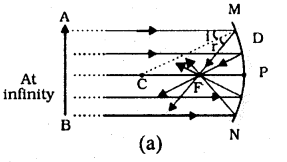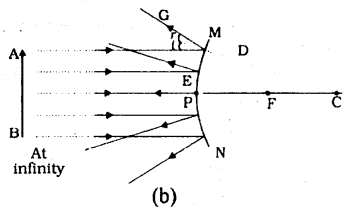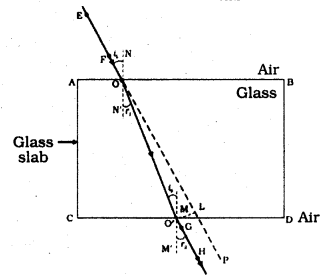## KSEEB Solutions for Class 9 Maths Chapter 3 Lines and Angles Ex 3.3

KSEEB Solutions for Class 9 Maths Chapter 3 Lines and Angles Ex 3.3 are part of KSEEB Solutions for Class 9 Maths. Here we have given Karnataka Board Class 9 Maths Solutions Chapter 3 Lines and Angles Exercise 3.3.

## Karnataka Board Class 9 Maths Chapter 3 Lines and Angles Ex 3.3

Question 1.
In Fig. 3.39, sides QP and RQ of ∆PQR are produced to points S and T respectively. If ∠SPR = 135° and ∠PQT = 110°, find ∠PRQ.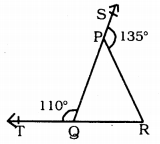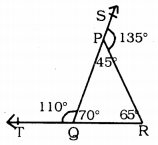Arms of the ∆PQR QP and RP are produced to S and T and ∠PQT = 110°, ∠SPR = 135°. ∠PRQ =?
Straight-line PR is on straight line SQ.
∠SPR and ∠RPQ are Adjacent angles.
∴ ∠SPR + ∠RPQ = 180°
135 + ∠RPQ = 180°
∴ ∠RPQ =180 – 135
∠RPQ = 45° (i)
Similarly QP straight line is on straight line TR.
∠TQP and ∠PQR are Adjacent angles.
∴ ∠RQP + ∠PQR = 180°
110 + ∠PQR = 180°
∠PQR = 180 – 110
∴ ∠PQR = 70°
Now, in ∆PQR,
∠QPR + ∠PQR + ∠PRQ = 180°
45 + 70 + ∠PRQ = 180°
115 + ∠PRQ = 180°
∠PRQ = 180 – 115
∴ ∠PRQ = 65°.

Reference Angle Calculator. The reference angle is defined as the smallest possible angle made by the terminal side of the given angle with the x-axis.

Question 2.
In Fig. 3.40, ∠X = 62°. ∠XYZ = 54°. If YO and ZO are the bisectors of ∠XYZ and ∠XZY respectively of ∆XYZ, find ∠OZY and ∠YOZ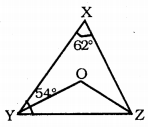In this figure, ∠X = 62°
∠XYZ = 54°
In ∆XYZ, YO and ZO are angular bisectors of ∠XYZ and ∠XZY.
Then, ∠OZY =?
∠OYZ =?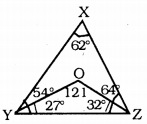In ∆XYZ
∠X + ∠Y + ∠Z = 180°
62 + 54 + ∠Z= 180
116 + ∠Z= 180
∠Z= 180- 116
∴ ∠Z = 64°
YO is the angular bisector of ∠Y
∴ ∠OYZ = $$\frac{54}{2}$$ = 27°
ZO is the angular bisector of ∠Z
∴ ∠OZY = $$\frac{64}{2}$$ = 32°
∴ ∠OZY = 32°
Now, in ∆OYZ,
∠OYZ + ∠OZY + ∠YOZ = 180°
27 + 32 + ∠YOZ = 180
59 + ∠YOZ = 180
∠YOZ = 180 – 59
∴∠YOZ = 121°
∴ ∠OZY = 32°
∠YOZ = 121°

Question 3.
In Fig. 3.41, if AB||DE, ∠BAC = 35° and ∠CDE = 53°, find ∠DCE.If AB || DE, ∠BAC = 35, ∠CDE = 53 then ∠DCE = ?
AB || DE, AE is the bisector.
∴∠BAC = ∠DEC = 35° (∵ Alternate angles)
∴∠DEC= 35°Now in ∆CDE,
∠DCE + ∠CDE + ∠CED = 180°
∠DCE + 53 + 35 = 180
∠DCE + 88 = 180
∠DEC = 180 – 88
∴ ∠DCE = 92°.

Question 4.
In Fig. 3.42, if lines PQ and RS intersect at point T, such that ∠PRT = 40°, ∠RPT = 95° and ∠TSQ = 75°, find ∠SQT.PQ and RS straight lines intersect at T.
If ∠PRT = 40°, ∠RPT = 95°, and ∠TSQ = 75°, then ∠SQT =?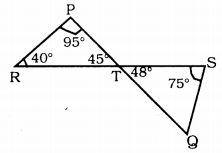In ∆PRT,
∠RPT + ∠PRT + ∠PTR = 180°
95 + 40 + ∠PTR = 180°
135 + ∠PTR = 180
∠PTR = 180 – 135
∴ ∠PTR = 45°
∠PTR = ∠STQ = 45° (∵ Vertically opposite angles)
In ∆TSQ,
∠STQ + ∠TSQ + ∠SQT =180
45 + 75 + ∠SQT = 180
120 + ∠SQT = 180
∴∠SQT = 180 – 120
∴ ∠SQT = 60°.

Question 5.
In Fig. 3.43, PQ ⊥ PS, PQ || SR, ∠SQR = 28° and ∠QRT = 65°. then find the value of x and y.PQ ⊥ PS, PQ ⊥ SR, ∠SQR = 28°, ∠QRT = 65°, Then x = ?, y = ?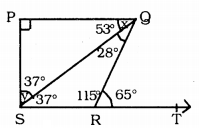Solution: ∠QRT + ∠QRS = 180° (∵ Linear pairs)
65 + ∠QRS = 180
∠QRS = 180 – 65
∴∠QRS =115°
In ∆SRQ,
∠QRS + ∠SQR + ∠RSQ = 180°
115 + 28 + ∠RSQ = 180
∴∠RSQ =180 – 143
∴∠RSQ = 37
Now, ∠RSQ = ∠PQS
37° = x
∴x = 37
In ∆SPQ,
∠SPQ + ∠PSQ + ∠PQS = 180°
90 + y + 37 = 180
∴y = 180- 127
∴y = 53°.

Question 6.
In Fig. 3.44, the side QR of ∆PQR is produced to a point S. If the bisectors of ∠PQR and ∠PRS meet at point T, then prove that ∠QTR = $$\frac{1}{2}$$∠QPR.Data: Arm QR of ∆PQR is produced upto S. Angular bisectors of ∠PQR and ∠PRS meet at T.
To Prove: ∠QTR = $$\frac{1}{2}$$ ∠QPR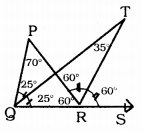Proof: Let ∠PQR = 50°, and ∠PRS = 120°
∠PQT = ∠TQR = 25°
∠PRT = ∠TRS = 60°
QR arm of ∆ PQR is produced upto S.
∴Exterior angle ∠PRS = ∠PQR + ∠QPR
120 = 50 + ∠QPR
∴ ∠QPR = 120 – 50
∠QPR = 70°
∴ ∠PRQ = 60°
Now, in ∆TRQ,
∠TQR + ∠TRQ + ∠QTR = 180°
25 + 120 + ∠QTR = 180°
145 + ∠QTR = 180°
∠QTR = 180 – 145
∴ ∠QTR = 35°
Now, ∠QTR = 35° ∠QPR = 70°
∠QTR = $$\frac{70}{2}$$
∴ ∠QTR = $$\frac{10}{2}$$ x ∠QPR.

We hope the KSEEB Solutions for Class 9 Maths Chapter 3 Lines and Angles Ex 3.3 help you. If you have any query regarding Karnataka Board Class 9 Maths Chapter 3 Lines and Angles Exercise 3.3, drop a comment below and we will get back to you at the earliest.

## KSEEB Solutions for Class 9 Maths Chapter 4 Polynomials Ex 4.2

KSEEB Solutions for Class 9 Maths Chapter 4 Polynomials Ex 4.2 are part of KSEEB Solutions for Class 9 Maths. Here we have given Karnataka Board Class 9 Maths Chapter 4 Polynomials Exercise 4.2.

## Karnataka Board Class 9 Maths Chapter 4 Polynomials Ex 4.2

Question 1.
Find the value of the polynomial 5x – 4x2 + 3 at
i) x = 0
ii) x = -1
iii) x = 2
i) f(x) = 5x – 4x2 + 3 x = 0 then,
f(0) = 5(0) – 4(0)2 + 3
=0 – 0 + 3
f(0) = 3

ii) f(x) = 5x – 4x2 + 3 x = -1 then,
f(-1) = 5(-1) – 4(-1)2 + 3
= -5 – 4(+1) + 3
= -5 – 4 + 3
= -9 + 3
f(-1) = -6

iii) f(x) = 5x – 4x2 + 3 x = 2 then,
f(2) = 5(2) – 4(2)2 + 3
= 5(2) – 4(4) + 3
= 10 – 16 + 3
= 13 – 16
f(2) = -3

Question 2.
Find p(0), p(1) and p(2) for each of the following polynomials :
i) p(y) = y2 – y + 1
ii) p(t) = 2 + t + 2t2 – t3
iii) p(x) = x3
iv) p(x) = (x – 1) (x + 1)
i) (a) p(y) = y2 – y + 1
p(0) = (0)2 – 0 + 1
= 0 – 0 + 1
∴ p(0) = =1

(b) p(y) = y2 – y + 1
p(1) = (1)2 – 1 + 1
= 1 – 1 + 1
∴ p(1) = 1

(c) p(y) = y2 – y +
p(2) = (2)2 – 2 + 1
= 4 – 2 + 1
= 5 – 2
∴ p(2) = 3

ii) (a) p(t) = 2 + t + 2t2 – t3
p(0) = 2 + 0 + 22 – (0)3
= 2 + 0 + 0 – 0
∴ p(0) = 2

(b) p(t) = 2 + t + 2t2 – t3
p(1) = 2 + 1 + 2(1)2 – (1)3
= 2 + 1 + 2(1) – 1
= 2 + 1 + 2 – 1
∴ p(1) = 4

(c) p(t) – 2 + t + 2t2 – t3
p(2) = 2 + 2 + 2(2)2 – (2)3
= 2 + 2 + 2(4) – 8
= 2 + 2 + 8 – 8
∴ p(2) = 4

iii) (a) p(x) = x3
p(0) = (0)3
p(0) = 0

(b) p(x) = x3
p(1) = (1)3
∴ p(1) = 1

(c) p(x) = x3
p(2) = (2)3
∴ p(2) = 8

iv) (a) p(x) = (x – 1)(x + 1)
p(x) = x2 – 1 [∵ (a + b)(a – b) = a2 – b]
p(0) = (0)2 – 1
= 0 – 1
∴ p(o) = -1

(b) p(x) = (x – 1)(x + 1)
p(x) = x2 – a [∵ (a + b)(a – b) = a2 – b2]
p(1) = (1)2 – 1
= 1 – 1
∴ p(1) = 0

(c) p(x) = (x – 1)(x + 1)
p(x) = x2 – 1 [∵ (a + b)(a – b) = a2 – b2]
p(2) = (2)2 – 1
= 4 – 1
∴ p(0) = 3

Question 3.
Verify whether the following are zeroes of the polynomial, induced against them.
i) p(x) = 3x + 1; $$x=-\frac{1}{3}$$
ii) p(x) = 5x – π; $$x=\frac{4}{5}$$
iii) p(x) = x2 – 1; x = 1, -1
iv) p(x) = (x + 1) (x – 2); x = -1, 2
v) p(x) = x2; x = 0
vi) p(x) = lx + m; $$x=-\frac{m}{l}$$
vii) p(x) = 3x2 – 1; $$x=-\frac{1}{\sqrt{3}}, \frac{2}{\sqrt{3}}$$
viii) p(x) = 2x + 1; $$x=\frac{1}{2}$$
i) p(x) = 3x + 1; $$x=-\frac{1}{3}$$Here value of polynomial is zero.
$$x=-\frac{1}{3}$$ is not zero of the polynomial

ii) p(x) = 5x – π; $$x=\frac{4}{5}$$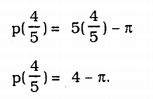Here value of polynomial is not zero.
$$x=\frac{4}{5}$$ is not zero of the polynomial

iii) p(x) = x2 – 1; x = 1, -1
p(1) = (1)2 – 1
= 1 – 1
p(1) = 0
Here value of p(x) is zero.
hence its zero is 1.
p(x) = x2 – 1; x = -1
p(-1) = (-1)2 – 1
= 1 – 1
p(-1) = 0
Here value of p(x) is zero.
∴ -1 is zero.

iv) p(x) = (x – 1)(x – 2); x = -1, 2
p(x) = x2 – 2x + x – 2
p(x) = x2 – x + 2 x = -1
p(-1) = (-1)2 – (-1)2 + 2
= 1 + 1 + 2
p(-1) = 4
Here value of polynomila is not zero.
∴ -1 is not zero.
p(x) = x2 – x + 2 x = 2
p(2) = (2)2 – (2) + 2
= 4 – 2 + 2
p(-1) = 4
Here value of polynomial is not zero.
∴ 2 is not zero.

(v) p(x) = x2; x = 0
p(0) = (0)2
p(0) = 0
Here value of p(x) is zero.
∴ 0 is its zero.

vi) p(x) = lx + m; $$x=-\frac{m}{l}$$
$$\mathrm{p}\left(-\frac{\mathrm{m}}{l}\right)=l\left(-\frac{\mathrm{m}}{l}\right)+\mathrm{m}$$
= -m + m
= 0
Here p(x) is zero.
∴ $$-\frac{\mathrm{m}}{l}$$ is its zero.

(vii) p(x) = 3x2 – 1; $$x=-\frac{1}{\sqrt{3}}, \frac{2}{\sqrt{3}}$$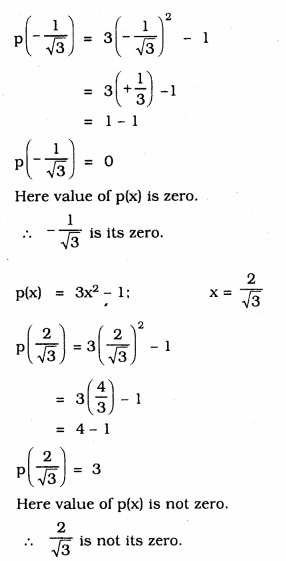viii) p(x) = 2x + 1; $$x=\frac{1}{2}$$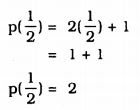Here value of p(x) is not zero.
∴ $$\frac{1}{2}$$ is not its zero.

Question 4.
Find the zero of the polynomial in each of the following cases :
(i) p(x) = x + 5
(ii) p(x) = x – 5
(iii) p(x) = 2x + 5
(iv) p(x) = 3x – 2
(v) p(x) = 3x
(vi) p(x) = ax, a ≠ 0
(vii) p(x) = cx + d, c ≠ 0, c, d are real numbers.
i) p(x) = x + 5
Let p(x) =0, then,
p(x) = x + 5 = 0
x = 0 – 5
∴ x = -5
-5 is zero of p(x).

ii) p(x) = x – 5
If p(x) = 0, then
p(x) = x – 5 = 0
x = 0 + 5
∴ x = 5
5 is the zero of p(x).

iii) p(x) = 2x + 5
If p(x)= 0, then
p(x) = 2x + 5 = 0
2x = – 5
∴ $$x=\frac{-5}{2}$$
$$\frac{-5}{2}$$ is the zero of p(x).

iv) p(x) = 3x – 2
If p(x)= 0, then
p(x) = 3x – 2 = 0
3x = 2
∴ $$x=\frac{2}{3}$$
$$\frac{2}{3}$$ is the zero of p(x).

v) p(x) = 3x
If p(x) = 0, then
p(x) = 3x = 0
∴ $$x=\frac{0}{3}$$
$$\frac{0}{3}$$ is the zero of p(x)

vi) p(x) = ax, a ≠ 0
If p(x)= 0, then
p(x) = ax = 0
∴ $$x=\frac{0}{a}$$ ∴ x = ∞(infinity)
∞ is the zero of p(x).

vii) p(x) = cx + d, c ≠ 0, c, d are real numbers
If p(x)= 0, then
p(x) = cx + d = 0
cx = 0 – d
cx = -d
∴ $$x=-\frac{d}{c}$$
$$-\frac{\mathrm{d}}{\mathrm{c}}$$ is the zero of p(x).

We hope the KSEEB Solutions for Class 9 Maths Chapter 4 Polynomials Ex 4.2 help you. If you have any query regarding Karnataka Board Class 9 Maths Chapter 4 Polynomials Exercise 4.2, drop a comment below and we will get back to you at the earliest.

## KSEEB Solutions for Class 9 Maths Chapter 4 Polynomials Ex 4.1

KSEEB Solutions for Class 9 Maths Chapter 4 Polynomials Ex 4.1 are part of KSEEB Solutions for Class 9 Maths. Here we have given Karnataka Board Class 9 Maths Chapter 4 Polynomials Exercise 4.1.

## Karnataka Board Class 9 Maths Chapter 4 Polynomials Exercise 4.1

Question 1.
Which of the following expressions are polynomials in one variable and which are not? State reasons for your answer.
i) 4x2 – 3x + 7
ii) y2 + $$\sqrt{2}$$
iii) $$3 \sqrt{t}+t \sqrt{2}$$
iv) $$\mathrm{y}+\frac{2}{\mathrm{y}}$$
v) x10 + y3 + t50
i) 4x2 – 3x + 7
Here polynomial has one variable, i.e. x
ii) y2 + $$\sqrt{2}$$
Here polynomial has one variable, i.e. y
iii) $$3 \sqrt{t}+t \sqrt{2}$$
This is polynmomial with one variable, because T is only one variable.
iv) $$\mathrm{y}+\frac{2}{\mathrm{y}}$$
Here polynomial has one variable, ie. y.
v) x10 + y3 + t50
This polynomial is not having one variable because here 3 variables means ‘x’, y and ‘t’ are there.

Question 2.
Write the coefficients of x in each of the followng :
i) 2 + x2 + x
ii) 2 – x2 + x3
iii) $$\frac{\pi}{2}$$x2 + x
v) $$\sqrt{2} \mathrm{x}$$ – 1
i) 2 + x2 + x
Here, coefficient of x2 is 1.
ii) 2 – x2 + x3
Here coefficient of x2 is -1
iii) $$\frac{\pi}{2}$$x2 + x
Here coefficient of x2 is $$\frac{\pi}{2}$$.
iv) $$\sqrt{2} \mathrm{x}$$ – 1
Here coefficint of x2 is -1.

Question 3.
Give one example each of a binomial of degree 35, and of a monomial of degree 100
i) A Bionomial of degree 35
E.g. f(x) = – x35 + 10
ii) A binomial of degree 100
E.g. f(y) = – y100.

Question 4.
Write the degree of each of the following polynomials :
i) 5x3 + 4x2 + 7x
ii) 4 – y2
iii) 5t – $$\sqrt{7}$$
iv) 3
i) 5x3 + 4x2 + 7x Highest power (degree) 3
ii) 4 – y2 Highest power degree) 2
iii) 5t – $$\sqrt{7}$$ Highest power (degree) 1
iv) 3 Highest power (degree) 0

Question 5.
Classify the folloiwng as linear, quadratic and cubic polynomials :
i) x2 + x
ii) x – x3
iii) y + y2 + 4
iv) 1 + x
iii) 3t
iv) r2
vii) 7x3

 Linear Polynomial Quadratic Polynomial Cubic Polynomial iv) 1 + x i) x2 + x iii) y + y2 + 4 ii) x – x3 (v) 3t (vi) r2 (vii) 7x3

We hope the KSEEB Solutions for Class 9 Maths Chapter 4 Polynomials Ex 4.1 help you. If you have any query regarding Karnataka Board Class 9 Maths Chapter 4 Polynomials Exercise 4.1, drop a comment below and we will get back to you at the earliest.

## KSEEB SSLC Class 10 Science Solutions Chapter 1 Chemical Reactions and Equations

KSEEB SSLC Class 10 Science Solutions Chapter 1 Chemical Reactions and Equations are part of KSEEB SSLC Class 10 Science Solutions. Here we have given Karnataka SSLC Class 10 Science Solutions Chapter 1 Chemical Reactions and Equations.

## Karnataka SSLC Class 10 Science Solutions Chapter 1 Chemical Reactions and Equations

### KSEEB SSLC Class 10 Science Chapter 1 Intext Questions

Text Book Part I Page No. 6

Question 1.
Why should a magnesium ribbon be cleaned before burning in air?
Magnesium metal is highly reactive. In stored conditions, it reacts with oxygen to form magnesium oxide over its outer layer. To remove this layer and to expose the underlying metal into air, the magnesium ribbon is cleaned by sandpaper.

Question 2.
Write the balanced equation for the following chemical reactions.
i) Hydrogen + Chlorine ➝ Hydrogen Chloride
H2(g) + Cl2(g) ➝ 2HCl(g)

ii) Barium chloride + Aluminium sulphate ➝ Barium sulphate + Aluminium chloride
3BaCl2(s) + Al2(SO4)3(s) ➝ 3BaSO4 + 2Al2Cl3(s)

iii) Sodium + water ➝ Sodium hydroxide + Hydrogen
2Na(s) + 2H2O(l) ➝ 2NaOH(aq) + H2(g)

Question 3.
Write a balanced chemical equation with state symbols for the following reactions.
i) Solutions of barium chloride and sodium sulphate in water react to give insoluble barium sulphate and the solution of sodium chloride.
Balanced chemical equations with state symbols for the required reactions are as follows:

1. BaCl2(aq) + Na2SO4(aq) → BaSO4(s) + 2NaCl(aq)
2. NaOH(aq) + HCl(aq) + NaCl(aq) + H2O(l)

Text Book Part I Page No. 10

Question 1.
A solution of a substance ‘X’ is used for whitewashing.
i) Name the substance ‘X’ and write its formula.
The substance X is calcium Hydroxide. Its formula is Ca(OH)2

ii) Write the reaction of the substance ‘X’ named in (i) above with water.
Cao + H2O ➝ Ca(OH)2

Question 2.
Why is the amount of gas collected in one of the test tubes in ActivityWater (H2O) contains two parts of hydrogen and one part of oxygen. The ratio of water components i.e., hydrogen and oxygen is 2:1. Therefore, the amount of hydrogen and oxygen produced after water electrolysis is in a ratio of 2:1. This is why during electrolysis the amount of gas collected in hydrogen’s test tubes is double the amount collected in the oxygen’s test tube.

1. Take a plastic mug. Drill two holes at its base and fit rubber stoppers in these holes. Insert carbon electrodes in these rubber stoppers as shown in Figure.
2. Connect these electrodes to a 6-volt battery.
3. Fill the mug with water such that the electrodes are immersed. Add a few drops of dilute sulphuric acid to the water.
4. Take two test tubes filled with water and invert them over the two carbon electrodes.
5. Switch on the current and leave the apparatus undisturbed for some time.
6. You will observe the formation of bubbles at both electrodes. These bubbles displace water in the test tubes.
7. Is the volume of the gas collected the same in both the test tubes?
8. Once the test tubes are filled with the respective gases. Remove them carefully.
9. Test these gases one by one by bringing a burning candle close to the mouth of the test tubes.

Caution:

1. This step must be performed carefully by the teacher.
2. What happens in each case?
3. Which gas is present in each test tube?

Text Book Part I Page No. 13

Question 1.
Why does the colour of copper sulphate solution change when an iron nail is dipped in it?
Iron is more reactive than copper. When an iron nail is dipped in copper sulphate solution, iron forms its sulphate (iron sulphate) solution by displacing copper of copper sulphate. The colour of iron sulphate is green. So, colour change in solution appears.

Question 2.
Give an example of a double displacement reaction other than the one given in Activity 1.10.
2KBr(aq)+BaI2(aq) ➝ 2KI(aq) + BaBr2 (aq)

Question 3.
Identify the substances that are oxidised and the substances that are reduced in the following reactions.
i) 4Na(s) + O2(g) ➝ 2Na2O(s) 4Na(s)
Sodium is oxidized because it gets oxygen and forms sodium oxide.

ii) CuO(s) + H2(g) ➝ Cu(s) + H2O(l)
Copper oxide reduced to copper H2 changes to water.

### KSEEB SSLC Class 10 Science Chapter 1 Textbook Exercises

Question 1.
Which of the statements about the reaction below are incorrect?
2PbO(s) + C(s) ➝ 2Pb(s) + CO2(g)
b) Carbon dioxide is getting oxidised.
c) Carbon is getting oxidised.
d) Lead oxide is getting reduced.
i) (a) and (b)
ii) (a) and (c)
iii) (a), (b) and (c)
iv) all
(i) (a) and (b).
Lead is getting reduced. & Carbon dioxide is getting oxidised.

Question 2.
Fe2O3 + 2Al ➝ Al2O3 + 2Fe
The above reaction is an example of a
a) combination reaction
b) double displacement reaction.
c) decompoistion reaction.
d) displacement reaction.
(d) The reaction is an example of a displacement reaction.

Question 3.
What happens when dilute hydrochloric acid is added to iron fillings? Tick the correct answer.
a) Hydrogen gas and iron chloride are produced.
b) Chlorine gas and iron hydroxide are produced.
c) No reaction takes place.
d) Iron salt and water are produced.
(a) Hydrogen gas and iron chloride are produced.
Fe(s) + 2HCl(aq) ➝ FeCl2(aq) + H2l

Question 4.
What is a balanced chemical equation? Why should chemical eqations be balanced?
The total mass of the elements present in the products of a chemical reaction has to be equal to the total mass of the elements present in the reactants. In other words, the number of atoms of each element remains the same, before and after a chemical reaction. This is called Balancing equation. We must balance the chemical equation, otherwise it becomes skeletal chemical equation.

Question 5.
Translate the following statements into chemical equations and then balance them.
(a) Hydrogen gas combines with nitrogen to form ammonia.
(b) Hydrogen sulphide gas burns in air to give water and sulphur dioxide.
(c) Barium chloride reacts with aluminium sulphate to give aluminium chloride and a precipitate of barium sulphate.
(d) Potassium metal reacts with water to give potassium hydroxide and hydrogen gas.
(a) 3H2(g) + N2(g) → 2NH3(g).
(b) 2H2S(g) + 3O2(g) → 2H2O(l) + 2SO2(g).
(c) 3BaCl2(aq) + Al2(SO4)3(aq) → 2AlCl3(aq) + 3BaSO4(s).
(d) 2K(s) + 2H2O(l) → 2KOH(aq) + H2(g).

Question 6.
Balance the following chemical equations.
a) HNO3 + Ca(OH)2 ➝ Ca(NO3)2 + H2O
b) NaOH + H2SO4 ➝ Na2SO4 + H2O
c) NaCl + AgNO3 ➝ AgCl + NaNO3
d) BaCl2 + H2SO4 ➝ BaSO4 + HCl
a) 2HNO3 + Ca(OH)2 ➝ Ca(NO3)2 + 2H2O
b) 2NaOH + H2SO4 ➝ Na2SO4 + 2H2O
c) NaCl + AgNO3 ➝ AgCl + NaNO3
d) BaCl2 + H2SO4 ➝ BaSO4 + 2HCl

Question 7.
Write the balanced chemical equations for the following reactions.
a) Calcium hydroxide + Carbon dioxide ➝ Calcium carbonate + Water
b) Zinc + Silver nitrate ➝ Zinc nitrate + Silver
c) Aluminium + Copper chloride ➝ Aluminium chloride + Copper
d) Barium chloride + Potassium sulphate ➝ Barium sulphate + Potassium chloride
a) Ca(OH)2 + CO2 ➝ CaCO3 + H2O
b) Zn + 2AgNO3 ➝ Zn(NO3)2 + 2Ag
c) 2Al + 3CuCl2 ➝ AlCl3 + 3Cu
d) BaCl2 + K2SO4 ➝ BaSO4 + KCl

Question 8.
Write the balanced chemical equation for the following and identify the type of reaction in each case.
a) Potassium bromide(aq) + Barium iodide(aq) ➝ Potassium iodide (aq) + Barium bromide(s)
b) Zinc carbonate(s) ➝ Zinc oxide(s) + Carbon dioxide(g)
c) Hydrogen(g) + Chlorine(g) ➝ Hydrogen chloride(g)
d) Magnesium(s) + Hydrocholoric acid(aq) ➝ Magnesium chloride(aq) + Hydrogen(g)
a) 2KBr(aq) + Bal2(aq) ➝ 2KI(aq) + BaBr2(s) ➝ double displacement reaction.
b) ZnCO3(s) ➝ ZnO(s) + CO2(g) ➝ decompoistion reaction.
c) H2(g) + Cl2(q) ➝ 2HCl(g) ➝ combination reaction
d) Mg(s) + 2HCl(aq) —> MgCl2(aq) + H2(g) ➝ displacement reaction.

Question 9.
What does one mean by exothermic and endothermic reactions? Give examples.

1.  Reactions in which heat is released along with the formation of products are called exothermic chemical reactions.
Eg: CH4(g)+2O2(g) ➝ CO2(g) + 2H2O(g)
2.  Reactions in which energy is absorbed are known as endothermic reactions.
6CO2 + 6H2O(l) ➝ C6H12O6(aq) + 6O2(g)

Question 10.
Why is respiration considered an exothermic reaction? Explain.
We get from the food we eat. During digestion complex molecules of food are broken into simpler molecule such as glucose. This glucose combines with oxygen in the cells of our body and provides energy. Therefore respiration is considered an exothermic reaction.

Question 11.
Why are decomposition reactions called the opposite of combination reactions? Write equations for these reactions.
Decomposition reactions involve breaking down of compounds to form two or more substances. These reactions require energy to proceed. Thus, they are the exact opposite of combination reactions in which two or more substances combine to give a new substance.

Examples:

1. ZnCO3(s) → ZnO(s) + CO2(g); Decomposition reaction.
2. H2(g) + Cl2(g) → 2HCl(g); Combination reaction.

In the first equation, since ZnCO3 is broken down into ZnO and CO2 it is a decomposition reaction. In the second equation, H2 and Cl2 combine to give a new substance HCl. Therefore, it is a combination reaction.

Question 12.
Write one equation each for decomposition reactions where energy is supplied in the form of heat, light or electricity.
Ans:
a) Thermal decompositionb) Decomposition by lightc) Decomposition by electricity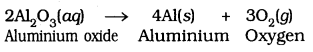Question 13.
What is the difference between displacement and double displacement reactions Write equations for these reactions.
In a displacement reaction, a more reactive element displaces a less reactive element from a compound.

A + BX → AX + B; where A is more reactive than B.
In a double displacement reaction, two atoms or a group of atoms switch places to form new compounds.
AB + CD → AD + CB
For example:
Displacement reaction:
CuSO4(aq) + Zn(s) → ZnSO4(aq) + Cu(s)

Double displacement reaction:
Na2SO4(aq) + BaCl2 (aq) → BaSO4(s) + 2NaCl(aq)

Question 14.
In the refining of silver, the recovery of silver from silver nitrate solution involved displacement by copper metal. Write down the reactions involved.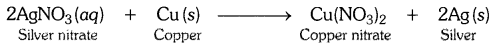Question 15.
What do you mean by a precipitation reaction? Explain by giving examples.
Any reaction that produces a precipitate can be called a precipitation reaction.
Eg:In this reaction Barium chloride obtained as precipitate.
The white precipatate of BaSO4 is formed by the reaction of SO42- and Ba2+. The other product is formed is Sodium Chloride which remains in the solution.

Question 16.
Explain the following in terms of gain or loss of oxygen with two examples each.
a) Oxidation (Gain of Oxygen)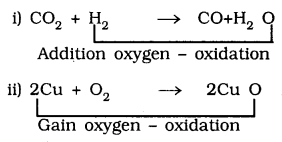In equation (i) From H2, H2O is oxidised. In eqn (ii) From Cu, CuO is oxidised
b) Reduction is loss of Oxygen:In eqn (i) CO2 is reduced to CO. in eqn (ii) CuO is reduced to Cu.

Question 17.
A shiny brown coloured element ‘X’ on heating in air becomes black in colour. Name the element ‘X’ and the black coloured compound formed.
‘X’ means copper (Cu) and black coloured compound copper oxideQuestion 18.
Why do we apply paint on iron articles?
Iron articles are painted to prevent them from rusting. When painted, the contact of iron articles with atmospheric moisture and the air is cut off. Hence, rusting is prevented.

Question 19.
Oil and fat containing food items are flushed with nitrogen. Why?

Oil and fat containing food items are flushed with nitrogen to prevent the items from getting oxidised which may result in rancidity of such products. When fats and oils are oxidised, they become rancid and their smell and taste change. Nitrogen provides an inert atmosphere for them.

Question 20.
Explain the following terms with one example each.
a) Corrosion
b) Rancidity

(a) Corrosion:
When a metal is attacked by substances around it such as moisture, acids etc. it gets corroded and the process is called corrosion. For example, rusting of iron products.

(b) Rancidity:
The Process in which fats and oils or food products made from fats or oils get oxidised resulting in a change of smell and the taste is called rancidity. For example, food items made from oil like chips becomes rancid if kept open for some time.

To prevent rancidity antioxidants (which prevent oxidation) are added to food containing fats and oils. Rancidity can also be prevented by flushing out oxygen with a gas like nitrogen. For example packets of food items like chips are flushed with nitrogen so that those can be used even after long duration.

### KSEEB SSLC Class 10 Science Chapter 1 Additional Questions and Answers

Question 1.
How do you determine whether a chemical reaction has taken place?
Following observations helps us to determine whether a chemical reaction has taken place

1.  Change in state
2.  Change in colour
3.  evolution of a gas
4.  Change in temperature.

Question 2.
Write the Balanced equation for the reaction of iron with steam.
3Fe(s) + 4H2O(g) ➝ Fe3O4(s) + 4H2(g)

Question 3.
What is quicklime? Write one use of quicklime.
Calcium oxide is called lime or quicklime. It is used in the manufacture of cement.

Question 4.
What are antioxidants?
The substances which prevent oxidation are called antioxidants.

We hope the given KSEEB SSLC Class 10 Science Solutions Chapter 1 Chemical Reactions and Equations will help you. If you have any query regarding Karnataka SSLC Class 10 Science Solutions Chapter 1 Chemical Reactions and Equations, drop a comment below and we will get back to you at the earliest.

## KSEEB SSLC Class 10 Science Solutions Chapter 16 Sustainable Management of Natural Resources

KSEEB SSLC Class 10 Science Solutions Chapter 16 Sustainable Management of Natural Resources are part of KSEEB SSLC Class 10 Science Solutions. Here we have given Karnataka SSLC Class 10 Science Solutions Chapter 16 Sustainable Management of Natural Resources.

## Karnataka SSLC Class 10 Science Solutions Chapter 16 Sustainable Management of Natural Resources

### KSEEB SSLC Class 10 Science Chapter 16 Intext Questions

Text Book Part I Page No. 128

Question 1.
What changes can you make in your habits to become more environment friendly?

1.  We must refuse to buy products that harm us and the environment.
2.  We should minimise the use of electricity and water.
3.  We should encourage recycling of things.

Question 2.
What would be the advantages of exploiting resources with short-term aims?
With the human population increasing at a tremendous rate due to improvement in health-care, thedem and for all resources is increasing at an exponential rate. The management of natural resources requires a long term perspective so that these will last for the generations to come and will not merely be exploited to the hilt for short term gains.

Question 3.
How would these advantages differ from the advantages of using a long term perspective in managing our resources?
If resources are used in accordance with short term aims, present generation will be able to utilize the resources properly for overall development. But if we plan to use resources with long term aims, not only the present generation is benefited but also the future generations will also be able to utilize resources for fulfilling its necessities. Thus it would be better to use our natural resources with a long term perspective so that it could be used by the present generation as well as conserved for future use.

Question 4.
Why do you think that there should be equitable distribution of resources? What forces would be working against an equitable distribution of our resources?
Nature shows no partiality. Natural resources belong to all and these resources should be used judiciously. Equitable distribution of resources will benefit both poor as well as rich people.
Human greed, corruption, and the lobby of the rich and powerful are the forces working against an equitable distribution of our resources.

Text Book Part I Page No. 132

Question 1.
Why should we conserve forests and wildlife?

1. Destruction of forests not only affect on forest products but it affects the water resources and it is soil pollution.
2. Destruction of forest leads to shortage of fodder for animals, shortage of medicinal plants, shortage of fruits and nuts.
3. There is a shortage of valuable timbers such as sal, sandal wood etc.
4. Wild animals are also useful to us in many ways, hence we should conserve these by building sanctuaries and prohibited hunting.

Question 2.
Suggest some approaches towards the conservation of forests.

1. Cutting valuable trees should be prevented.
2. We should use forest products, such that there should not be damage to the environment.
3. All people should participate in the conservation of forest and wild animals.

Text Book Part I Page No. 135

Question 1.
We must dug small pits and lakes, put in place simple water shed systems, built small earthen dams, constructed dykes, sand and limestone reservoirs, set up root top water collecting units. These are the traditional systems of water harvesting/management in our region.

Question 2.
Compare the above system with the probable systems in hilly/ mountainous areas or plains or plateau regions.
In the above mentioned places check dams are built because here water harvesting is difficult.

Question 3.
Find out the source of water in your region/locality. Is water from this source available to all people living in that area?
Tube wells and river water (Tungabhadra) are the water sources available to all people in our area. There are different sources in different places. In some places there is too much shortage of water because of failure of rain recently.

### KSEEB SSLC Class 10 Science Chapter 16 Textbook Exercises

Question 1.
What changes would you suggest in your home in order to be environment-friendly?

1. We must save water and electricity.
2. We should not waste food.
3. We should encourage reuse and recycling.
4. We must minimise the use of plastics.

Question 2.
Can you suggest some changes in your school which would make it environment friendly?

1. Enough plants and trees can be planted in the school.
2. Water should not be wasted but should be used judiciously.
3. Students should be taught to keep their classrooms and immediate surroundings neat and tidy.
4. Compost pits may be made in safe comers of the school, where biodegradable wastes may be dumped to prepare compost.
5. Minimising the usage of loudspeakers.
6. Organising seminars, quiz, essay competition, drawing competitions for spreading environmental awareness, celebrating Vanamahotsava… etc.

Question 3.
We saw in this chapter that there are four main stakeholders when it comes to forests and wildlife. Which among these should have the authority to decide the management of forest produce? Why do you think so?
The local people need large quantities of firewood, small timber and thatch. Bamboo is used to make slats for huts, and baskets for collecting and storing food materials. Implements for agriculture, fishing and hunting are largely made of wood, also forests are sites for fishing and hunting.

In addition to the people gathering fruits, nuts and medicines from the forests, there cattle also graze in forest areas or food on the fodder which is collected from forests.

Because of these reasons the people who live in or around forests have authority to decide the management of forest produce.

Question 4.
How can you as an individual contribute or make a difference to the management of
(a) forests and wildlife
(b) water resources and
(c) coal and petroleum?
a) Forests and wild animals.

1. cutting valuable trees should be avoided by destroying forest affects the quality of soil and water resources.
2. Hunting should be prohibited.
3. There should be wild sanctuaries which gives protection for wild animals.

b) Water Resources:

1. Water resources should be free from pollution.
2. Excess usage of water should be avoided.

c) Coal and Petroleum:
We should minimise the use of coal and petroleum, because these are fossil fuels. By burning these there are ill effects such as air pollution and acid rainfall etc.

Question 5.
What can you as an individual do to reduce your consumption of the various natural resources?

1. We must have come across the five R’s to save the environment: Refuse, Reduce, Reuse, Repurpose and Recycle.
2. We should encourage tree plantation programmes.
3. We must reduce the burning of fossil fuels.
4. Encouragement should be given for harvesting the water.

Question 6.
List five things you have done over the last one week to —
(a) conserve our natural resources.
We should travel in bus instead of using own vehicles or we should practice walking, we must use LED bulbs or fluorescent tubes in our homes. We must use the lift or taking the stairs, wearing an extra sweater or using a heating device (heater or sign) on cold days.

(b) increase the pressure on our natural resources.

1. We should grow Number of trees around our house.
2. Reducing own vehicles by using public transport system or by, walking.
3. There should not be more factories.
4. We must prevent soil erosion.
5. We must reduce the usage of vehicles to avoid air pollution.

Question 7.
On the basis of the issues raised in this chapter, what changes would you incorporate in your lifestyle in a move towards sustainable use of our resources?
We need to change our lifestyles so that we can use natural resources on a sustainable basis. The changes which can be brought about are as follows:

• Stop cutting trees and start planting trees.
• Use LED bulbs and fluorescent tubes.
• Take the stairs and avoid using lifts.
• During summers use bamboo made fans avoid air coolers and electricians.
• Use more of public transport.
• Let our conscience be always alert not to pollute the environment from any of our activities.

### KSEEB SSLC Class 10 Science Chapter 16 Additional Questions and Answers

1. Fill in the blanks

Question 1.
…… and …… are followed as means of protection of nature and natural resources.

Question 2.
Prticipation of the …… can indeed lead to the efficient management of forests.
local people

Question 3.
Irrigation methods like have been used in various parts of India since ancient times.
dams, tanks and canals.

Question 4.
…… and …… were formed from the degradation of biomass millions of years ago.
Coal, petroleum

Question 5.
Fossil fuels contain carbon along with …… also.
hydrogen, nitrogen and sulphur.

We hope the given KSEEB SSLC Class 10 Science Solutions Chapter 16 Sustainable Management of Natural Resources will help you. If you have any query regarding Karnataka SSLC Class 10 Science Solutions Chapter 16 Sustainable Management of Natural Resources, drop a comment below and we will get back to you at the earliest.

## KSEEB SSLC Class 10 Science Solutions Chapter 15 Our Environment

KSEEB SSLC Class 10 Science Solutions Chapter 15 Our Environment are part of KSEEB SSLC Class 10 Science Solutions. Here we have given Karnataka SSLC Class 10 Science Solutions Chapter 15 Our Environment.

## Karnataka SSLC Class 10 Science Solutions Chapter 15 Our Environment

### KSEEB SSLC Class 10 Science Chapter 15 Intext Questions

Text Book Part I Page No. 140

Question 1.
What are the trophic levels? Give an example of a food chain and state the different trophic levels in it.
Each step or level of a food chain is called Trophic levels.
Example for Food chainHere grass is a producer because it prepares its own food. This grass is eaten by herbivores means secondary, small carnivores (Frog) are tertiary and higher carnivores are in the fourth level.

Question 2.
What is the role of decomposers in the ecosystem?
Microorganisms, comprising bacteria and Fungi, break-down the dead remains and waste products of organisms. These microorganisms are the decomposers as they break-down the complex organic substances into simple inorganic substances that go into the soil and are used up once more by the plants.

Text Book Part I Page No. 142

Question 1.
Substances that are broken down by biological processes are said to be biodegradable. Eg: paper and peel of a fruit. But plastic leather etc. are not broken down. These are called Non-biodegradable.

Question 2.
Give any two ways in which biodegradable substances would affect the environment.

1. Leaves of the plants decay and reduces soil fertility,
2. Bio-degradable substances have carbon. When this is burnt, CO2 and CO are produced and causes air pollution.

Question 3.
Give any two ways in which non-biodegradable substances would affect the environment.

1. As these are not decomposing, they cause air pollution and water pollution.
2. Plastic enters stomach of many animals and causes death of animals.

Text Book Part I Page No. 144

Question 1.
What is ozone and how does it affect any ecosystem?
Ozone at the higher levels of the atmosphere is a product of UV radiations acting on O2 molecule. The higher energy UV radiations split apart some molecular Oa in free oxygen (O) atoms. These atoms then combine with the molecular O2 to form Ozone.Ozone shields the surface of the earth from ultraviolet (UV) radiation from the Sun. This radiation is highly damaging to organisms for example, it is known to cause skin cancer in human beings.

Question 2.
How can you help in reducing the problem of waste disposal? Give any two methods.

1. We must minimise the usage of plastics,
2. We can collect wastes and by this we can produce gas which is an alternate source of energy.

### KSEEB SSLC Class 10 Science Chapter 15 Textbook Exercises

Question 1.
Which of the following groups contain only biodegradable items?
(a) Grass, flowers and leather.
(b) Grass, wood and plastic.
(c) Fruit-peels, cake and lime-juice.
(d) Cake, wood and grass.
(b) Grass, wood and plastic.

Question 2.
Which of the following constitute a food-chain?
(a) Grass, wheat and mango.
(b) Grass, goat and human.
(c) Goat, cow and elephant.
(d) Grass, fish and goat.
(b) Grass, goat and human.

Question 3.
Which of the following are environment-friendly practices?
(a) Carrying cloth-bags to put purchases in while shopping.
(b) Switching off unnecessary lights and fans.
(c) Walking to school instead of getting your mother to drop you on her scooter
(d) All of the above.
(d) All of the above.

Question 4.
What will happen if we kill all the organisms in one trophic level?
If we kill all the organisms in one trophic level, the population size of organisms in lower level increases uncontrollably and the number of organisms in higher trophic level decreases due to non¬availability of food. This results in an imbalance in ecosystem.

Question 5.
Will the impact of removing all the organisms in a trophic level be different for different trophic levels? Can the organisms of any trophic level be removed without causing any damage to the ecosystem?

• Removing producers: All the heterotrophs die.
• Removing herbivores: Carnivores would not get food.
• Removing carnivores: Herbivores would increase to unsustainable levels.
• Removing decomposers: Organic wastes, plant, and animal dead remains would pile up.
• The role of each and every species belonging to every trophic level is unique.
• No, the organisms of any trophic level cannot be removed without damaging the ecosystem.

Question 6.
What is the biological magnification? Will the levels of this magnification be different at different levels of the ecosystem?
Some harmful chemicals enter our bodies through the food chain, one of the reasons is the use of several pesticides and other chemicals to protect our crops from disease and pests. These chemicals are either washed down into the soil or into the water bodies. From the soil, these are absorbed by the plants along with water and minerals and from the water bodies these are taken up by aquatic plants and animals.

This is one of the ways in which they enter the food chain. This phenomenon is known as biological magnification. This level of magnification be different at different levels of the ecosystem.

Eg: Spraying of DDT will remain for a long time in the environment.

Question 7.
What are the problems caused by the non-biodegradable wastes that we generate?

• Non-aesthetic look.
• Death of cattle by ingestion of plastic bags.
• The quality of soil is adversely affected.
• Biomagnification of harmful chemicals like DDT in birds disturb their calcium metabolism.
• Non – biodegradable wastes cause pollution of soil and water.

Question 8.
If all the waste we generate is biodegradable, will this have no impact on the environment?
If all the waste we generate is biodegradable, there is a imbalance in nature. Because with the increase of wastes there is decrease in the number of decomposers. These wastes spread every where and microbes are more which causes many diseases to us.

Question 9.
Why is damage to the ozone layer a cause for concern? What steps are being taken to limit this damage?
Damage to the ozone layer causes so many problems. At the higher levels of the atmosphere, ozone performs an essential function. It shields the surface of the earth from ultraviolet radiation from the sun. If ozone layer is damaged no organism can survive. The following are the steps being taken to limit this damage.

1. We should minimize the use of vehicles.
2. We should not encourage the burning of fossilic fuels.
3. It is now mandatory for all the manufacturing companies to make CFC- free refrigerators throughout the world.

We hope the given KSEEB SSLC Class 10 Science Solutions Chapter 15 Our Environment will help you. If you have any query regarding Karnataka SSLC Class 10 Science Solutions Chapter 15 Our Environment, drop a comment below and we will get back to you at the earliest.

## KSEEB SSLC Class 10 Science Solutions Chapter Chapter 14 Sources of Energy

KSEEB SSLC Class 10 Science Solutions Chapter 14 Sources of Energy are part of KSEEB SSLC Class 10 Science Solutions. Here we have given Karnataka SSLC Class 10 Science Solutions Chapter 14 Sources of Energy.

## Karnataka SSLC Class 10 Science Solutions Chapter 14 Sources of Energy

### KSEEB SSLC Class 10 Science Chapter 14 Intext Questions

Text Book Part I Page No. 110

Question 1.
What is a good source of energy?
We could then say that a good source of energy would be one.

1. Which would do a large amount of work per unit volume or mass.
2. be easily accessible.
3. be easy to store and transport, and
4. perhaps most importantly, be economical.

Question 2.
What is a good fuel?
A good fuel is one which

• produces more heat per unit mass. It has high calorific value.
• produces less harmful gases on combustion.
• is cheap and easily available.
• is every to handle safe to transport and convenient to store.

Question 3.
If you could use any source of energy for heating your food, which one would you use and why?
We should select which is easily available and it should be cheaper. Bio-gas is an excellent fuel as it contains. It burns without some. Its heating capacity is high. This gas is convenient for consumption and transportation.

Text Book Part I Page No. 115

Question 1.
What are the disadvantages of fossil fuels?

• The fossil fuels are non renewable source of energy. If we were to consuming these sources as such an  alarming rate, we would soon run out of energy.
• Air pollution is. caused bybuTnmg fossil fuels.
• The oxides of carbon, nitrogen and ‘ sulphur drat are released on burning fossil fuels am acidic oxides. This lead to acid rain which affects our water and soil resources.
• Carbon dioxide produced by burning these fuels produces green house effect

Question 2.
Why are we looking at alternate sources of energy?
Fossil fuels are a non-renewable source of energy. So we need to conserve them. If we were to continue consuming these sources at such alarming rates, we would soon run out of energy. In order to avoid this, alternate sources of energy were explored.

Question 3.
How has the traditional use of wind and water energy been modified for our convenience?
(1) Wind energy: The kinetic energy of the wind can be used to do work. This energy was harnessed by wind mills in the past to do mechanical work. For example in a water lifting pump, the rotatory motion of windmill is utilized to lift water from a well. Today wind energy is also used to generate electricity. A windmill essentially consists of a structure similar to a large electric fan that is erected at some height on a rigid support.

A number of windmills are erected over a large area, which is known as wind energy farm. The energy output of each windmill in a farm is coupled together to get electricity on a commercial scale wind energy farms can be established only at those places where wind blows for the greater part of a year. The wind speed should also be higher than 15 km/h to maintain the required speed of the turbine, since, the tower and blades are exposed to the vagaries of nature like rain, sun, storm and cyclone, they need a high level of maintenance.

(2) Water energy: In order to produce hydel electricity, high rise dams are constructed on the river to obstruct the flow of water and thereby collect water in larger reservoirs. The water level rises and in this process the kinetic energy of flowing water gets transformed into potential energy. The water from the high level in the dam is carried through pipes, to the turbine, at the bottom of the dam. Sine the water in the reservoir would be refilled each time. It rains (hydropower is a renewable source of energy) we would not have to worry about hydro electricity sources getting used up the way fossil fuels would get finished one day.

Text Book Part I Page No. 120

Question 1.
What kind of mirror – concave, convex or plain – would be best suited for use in a solar cooker? Why?
A concave mirror would be best suited for use in a solar cooker. When a concave mirror reflector is. attached to a solar; cooker, it converges a large amount of sun’s be radiations at its focus due to which a high temperature is produced at die focus area.

Question 2.
What are the limitations of the energy that can be obtained from the oceans?
Tidal energy wave energy and ocean thermal energy are the sources of the energy that can be obtained from the oceans. Their limitations are as follows:

1. Tidal energy: The locations where such dams can be built are limited.
2. Wave energy: Wave energy would be a viable proposition only where waves are very strong.
3. Ocean thermal energy: Efficient commercial exploitation is difficult for this energy.

Question 3.
What is geothermal energy?
Due to geological changes, molten formed in the deeper hot regions of earth’s crust are pushed upward and trapped in certain regions called ‘hot spots’. This is called Geothermal energy.

Question 4.
What are the advantages of nuclear energy?

• It produces huge amount of energy form very small amount of a nuclear’ fuel.
• It does not produce gases like carbon 2. dioxide which contribute to green house effect or sulphur dioxide which causes acid rain.
• Once the nuclear fuel is loaded into the reaction, the nuclear power plant can go on producing electricity for 3. two to three years at a stretch. Hence, there is no need for putting in the nuclear fuel again and again.

Text Book Part I Page No. 120

Question 1.
Can any source of energy be pollution-free? Why or why not?
No source of energy be pollution free. Because fossil fuels cause air pollution. Nuclear energy causes more hazards for environment. In some cases, the actual operation of a device like the solar cell may be pollution-free, but the assembly of the device would have caused some environmental damage.

Question 2.
Hydrogen has been used as a rocket fuel. Would you consider it a cleaner fuel than CNG? Why or why not?
Hydrogen gas burns and produce steam. Because of this environment is not polluted. Hence it is used in rockets as fuel. But this gas is explosive. It is not a cleaner fuel than CNG. Because CNG is cleaner than the other sources of energy.

Text Book Part I Page No. 121

Question 1.
Name two energy sources that you would consider to be renewable. Give reasons for your choices.
Solar energy and wind energy are the two renewable sources of energy.
i) Solar energy: The sun has been radiating an enormous amount of energy at the present rate for nearly 5 billion years and will continue radiating at that rate for about 5 billion year more. A large number of solar cells are, combined in an arrangement called solar cell panel that can deliver enough electricity for practical use.

The principal advantages associated with solar cells are that they have no moving parts, require little maintenance and work quite ossificatory without the use of any focussing device. Another advantage is that they can-be set up in remote and in accessible hamlets or very sparsely inhabited areas in which laying of a power transmission line may be expensive and not commercially viable.

ii) Wind energy: Unequal heating of the landmass and water bodies by solar radiation generates air movement and causes winds to blow. This kinetic energy of the wind can be used to work. Wind energy is an environment-friendly and efficient source of renewable energy. It requires no recurring expenses for the production of electricity.

Question 2.
Give the names of two energy sources that you would consider to be exhaustible. Give reasons for your choices.

• fossil fuels
• Nuclear fuels

Fossil fuels are present in a limited. amount in the earth. Once exhausted, they will not be available to us again. It takes millions of years for fossil fuel to be formed. The nuclear materials which can be conveniently extracted from earth 7. are limited and hence they will get exhausted one day.

### KSEEB SSLC Class 10 Science Chapter 14 Textbook Exercises

Question 1.
A solar water heater cannot be used to get hot water on
(a) a sunny day
(b) a cloudy day
(c) a hot day
(d) a windy day
(b) a cloudy day.

Question 2.
Which of the following is not an example of a bio-mass energy source?
(a) wood
(b) gobar-gas
(c) nuclear energy
(d) coal
(c) nuclear energy.

Question 3.
Most of the sources of energy we use represent stored solar energy. Which of the following is not ultimately derived from the Sun’s energy?
(a) geothermal energy
(b) wind energy
(c) nuclear energy
(d) bio-mass.
(b) wind energy.

Question 4.
Compare and contrast fossil fuels and the Sun as direct sources of energy.
Air pollution is caused by burning of coal or petroleum products. The oxides of carbon, nitrogen and sulphur that are released on burning fossil fuels are acidic oxides. These lead to acid rain which affects our water and soil resources. In addition to the problem of air pollution, recall the green house effect of gases like carbon dioxide.

Sun is the main source Of energy, we get all sources of energy by solar energy. Hence this a renewable source of energy which we get in plenty and free of cost.

Question 5.
Compare and contrast bio-mass and hydro electricity as sources of energy.
Bio-mass: Cow-dung, various plant materials like the residue after . harvesting the crops, vegetable waste and sewage are decomposed in the absence of oxygen to give biogas. Since the starting material is mainly cow-dung, it is popularly known as ‘gober gas’. Biogas is produced in biogas plant.

Bio gas is an excellent fuel as it contains up to 75% Methane. It burns without smoke, leaves no residue like ash in wood, charcoal and coal burning. The slurry left behind is removed periodically and used as excellent manure. This is one of the renewable source of energy.

Hydro Electricity: In order to produce hydel electricity, high rise dams are constructed on the river to obstruct the flow of water and thereby collect water level rises and in this process the kinetic energy of flowing water, gets transformed into potential energy. The water from the high level in the dam is carried through pipes to the turbine, at the bottom of the dam. Since the water in the reservoir would be refilled each time it rains. We would not have to worry about hydro electricity sources getting used up the way fossil fuels would get finished one day.

Question 6.
What are the limitations of extracting energy from—
(a) the wind?
(b) waves?
(c) tides?
(a) The wind:

1. Wind energy farms can be established only at those places where wind blows for the greater part of a year.
2. The wind speed should also be higher than 15 km/h to maintain the required speed of the turbine.
3. Establishment of wind energy farms require large are of land.

These are the limitations of extracting energy from the wind.

(b) Limitations of extracting waves energy: The waves are generated by strong winds blowing across the sea. Wave energy would be a viable proposition only where waves are very strong.

(c) Limitations of extracting tidal energy: The locations where such dams can be built are limited.

Question 7.
On what basis would you classify energy sources as
(a) renewable and non-renewable?
(b) exhaustible and inexhaustible?
Are the options given in (a) and (b) the same?
The options given in (a) and (b) are the same.

Question 8.
What are the qualities of an ideal source of energy?
The qualities of an ideal source of energy are as follows:

1. Which would do a large amount of work per unit volume or mass.
2. Be easily accessible.
3. Be easy to store and transport, and
4. Perhaps most importantly, be economical.

Question 9.
What are the advantages and disadvantages of using solar cooker? Are there places where solar cookers would have limited utility?
Solar cookers have limited utility at places which remain cloudy or have larger winters eg, hilly areas. Advantages of using a solar cooker

• It cooks food without causing any kind of pollution.
• It is economical to use solar cooker because nothing is to be paid for using solar energy.
• It is easy to handle solar cooker and there is no chance of any kind of accident.
• It nutrients in the food do not get destroyed.

Disadvantages of using a solar cooker.

• Solar cooker cannot be used at night and during cloudy weather.
• It takes more time to cook food.
• The direction of solar cooker is to be changed

Question 10.
What are the environmental consequences of the increasing demand for energy? What steps would you suggest to reduce energy consumption?
They are:

• Burning of fossil fuels to meet the increasing demand for energy causes air pollution.
• Construction of dams and rivers to generate hydroelectricity destroys large ecosystems which get submerged underwater in the dams further, a large amount of methane [which is a green house gas] is produced when submerged vegetation rots under anaerobic conditions.

In order to reduce energy consumption

• Fossil fuel should be used with care and caution to derive maximum benefit out of them.
• Fuel saving devices such as pressure cookers etc should be used.
• Efficiency of energy sources should be maintained be getting them regularly serviced.
• And last of all, we should be economical in our energy consumption as energy saved is energy produced.

### KSEEB SSLC Class 10 Science Chapter 14 Additional Questions and Answers

Question 1.
Draw a neat diagram showing a model to demonstrate the process of thermoelectric production and label the parts.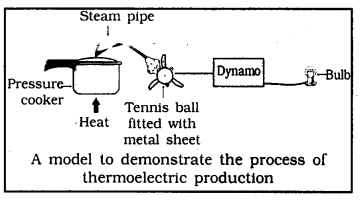Question 2.
Draw a neat diagram of a solar cooker and label the parts.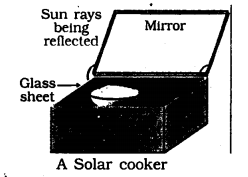We hope the given KSEEB SSLC Class 10 Science Solutions Chapter 14 Sources of Energy will help you. If you have any query regarding Karnataka SSLC Class 10 Science Solutions Chapter 14 Sources of Energy, drop a comment below and we will get back to you at the earliest.

## KSEEB SSLC Class 10 Maths Solutions Chapter 14 Probability Ex 14.1

KSEEB SSLC Class 10 Maths Solutions Chapter 14 Probability Ex 14.1 are part of KSEEB SSLC Class 10 Maths Solutions. Here we have given Karnataka SSLC Class 10 Maths Solutions Chapter 14 Probability Exercise 14.1.

## Karnataka SSLC Class 10 Maths Solutions Chapter 14 Probability Exercise 14.1

Question 1.
Complete the following statements :

1. Probability of an event E + Probability of the event ‘not E’ = _____
2. The Probability of an event that cannot happen is _____. Such an event is called ____.
3. The probability of an event that is certain to happen is ____. Such an event is called ____.
4. The sum of the probabilities of all the elementary events of an experiment is ____.
5. The probability of an event is greater than or equal to ____ and less than or equal to ____.

1. 1 : Probability of an event E + Probability of the event ‘not E’ = 1.
2. 0, impossible: The probability of an event that cannot happen is 0. Such an event is called impossible event.
3. 1, certain: The probability of an event that is certain to happen is 1. Such an event is called a sure or certain event.
4. 1: The sum of the probabilities of all the elementary events of an experiment is 1.
5. 0, 1: The probability of an event is greater than or equal to 0 and less than or equal to 1.

Question 2.
Which of the following experiments have equally likely outcomes? Explain.
(i) A driver attempts to start a car. The car starts or does not start.
(ii) A player attempts to shoot a basketball. She/he shoots or misses the shot.
(iv) A baby is born. It is a boy or a girl.
Solution:
(i) The outcome is not equally likely because the car starts normally only when there is some defect, the car does not start.
(ii) The outcome is not equally likely because the outcome depends on the training of the player.
(iii) The outcome in the trial of true-false question is, either true or false. Hence, the two outcomes are equally likely.
(iv) A baby can be either a boy or a girl and both the outcomes have equally likely chances.

Question 3.
Why is tossing a coin considered to be a fair way of deciding which team should get the ball at the beginning of a football game?
Because Tossing a coin considered to be a fair way of deciding which team should get the ball at the beginning of a football game. By this coin may be head or tail (only one). It has an equally likely outcome.

Question 4.
Which of the following cannot be the probability of an event?
A) $$\frac{2}{3}$$
B) -1.5
C) 15%
D) 0.7.
B) -1.5; This cannot be the probability of an event because possibility of event should not be less than 0 and more than 1. Hence -1.5 is lesser than 0.

Question 5.
If P(E) = 0.05, what is the probability of ‘not E’ ?
Solution:
IfP(E) = 0.05, then P($$\overline{\mathrm{E}}$$)=?
But, P(E) + P($$\overline{\mathrm{E}}$$) = 1 .
∴ P($$\overline{\mathrm{E}}$$) = 1 – 0.05
∴ P($$\overline{\mathrm{E}}$$) = 0.95.

Question 6.
A bag contains lemon flavoured candies only. Malini takes out one candy without looking into the bag what is the probability that she takes out.
(i) an orange flavoured candy?
(ii) a lemon flavoured candy?
Solution:
(i) Bag has only lemon flavoured candies. It has no orange candies.
∴ Possibility, P(E) = 0.
(ii) A lemon flavoured candy is possible. Because Bag contains all lemon flavoured candies.
∴ Possibility, P(F) = 1.

Question 7.
It is given that in a group of 3 students, the probability of 2 students not having the same birthday is 0.992. What is the probability that the 2 students have the same birthday?
Solution:
Let P(E) be an event 2 students have the same birthday i.e P(E) =?
P($$(\bar{E})$$) is an event 2 students not have same birth day P($$(\bar{E})$$) = 0.992
P(E) + P($$(\bar{E})$$) = 1
P(E) = 1 – P($$(\bar{E})$$) = 1.000 – 0.992 = 0.008
The probability of 2 students having the same birthday is 0.008.

Question 8.
A bag contains 3 red balls and 5 black balls. A ball is drawn at random from the bag. What is the probability that the ball drawn is (i) red? (ii) not red ?
Solution:
A bag contains 3 red balls, 5 black balls. Totally there are 8 balls.
∴ n(S) = 8
i) Possibility that the red ball drawn,
n(E) = 3
∴ Probability, P(E) = $$\frac{n(E)}{n(S)}=\frac{3}{8}$$
ii) Possibility that the 1 black ball drwn is
n(F) = 5
∴ Probability, P(F) = $$\frac{n(F)}{n(S)}=\frac{5}{8}$$

Question 9.
A box contains 5 red marbles, 8 white marbles and 4 green marbles. One marble is taken out of the box at random. What is the probability that the marble taken out will be
(i) red ?
(ii) white ?
(iii) not green ?
Solution:
A box contains 5 red marbles, 8 white marbles and 4 green marbles.
∴ Total number of marbles, n(S)
= 5 + 8 + 4
= 17
(i) Probability that the 1 red marble drawn is n(A) = 5
∴ Probability, P(A) = $$\frac{n(A)}{n(S)}=\frac{5}{17}$$
(ii) Possibility that 1 white marble drawn, n(B) = 8
∴ Probability, P(B) = $$\frac{n(B)}{n(S)}=\frac{8}{17}$$
(iii) Possibility that 1 not green marble ?
P(C) = 17 – 4 = 13 (∵ Except 4 green marbles)
∴ Probability, P(C) = $$\frac{n(C)}{n(S)}=\frac{13}{17}$$

Question 10.
A piggy bank contains hundred 50p coins, fifty Re. 1 coins, twenty Rs. 2 coins and ten Rs. 5 coins. If it is equally likely that one of the coins will fall out when the bank is turned upside down, what is the probability that the coin
(i) will be a 50 p coin ?
(ii) will not be a Rs. 5 coin ?
Solution:
Number of 50 ps coins = 100
Number of Re. 1 coins = 50
Number of Rs. 2 coins = 20
Number of Rs. 5 coins = 10
∴ Total number of coins, n(S) = 180
(i) Possibility of one 50 ps coin :
n(A) = 100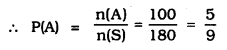(ii) Possibility of one Rs. 5 coin:
n(B) = 180 – 10 = 170
(∵ 10 coins are Rs. 5)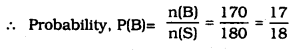Question 11.
Gopi buys a fish from a shop for his aquarium. The shopkeeper taks out one fish at random from a tank containing 5 male fish and 8 female fish (see figure given)What is the probability that the fish taken out is a male fish ?
Solution:
Number of male fish = 5
Number of female fish = 8
∴ Total number of fish, n(S) = 5 + 8 = 13
Probability that the fish taken out is male,
n(E) = 5Question 12.
A game of chance consists of spinning an arrow which comes to rest pointing at one of the numbers 1, 2, 3, 4, 5, 6, 7, 8 (see the Figure) and these are equally likely outcomes.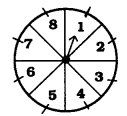What is the probability that it will point at
(i) 8?
(ii) an odd number
(iii) a number greater than 2 ?
(iv) a number less than 9 ?
Solution:
Total numbers in the spinning = 8
∴ n(A) = 1
(i) Possibility that points one number is 8 : n(A) = 1(ii) Possibility that points one odd number : 1, 3, 5, 7
∴ n(B) = 4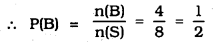(iii) A number greater than 2 :
3, 4, 5, 6, 7, 8
∴ n(C) = 6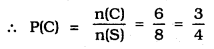(iv) A number less than 9 :
1, 2, 3, 4, 5, 6, 7, 8
∴ n(D) = 8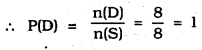Question 13.
A die thrown once. Find the probability of getting
(i) a prime number;
(ii) a number lying between 2 and 6;
(iii) an odd number.
Solution:
Possible event when a die is thrown:
1, 2, 3, 4, 5, 6
∴ n(S) = 6
(i) Probability of getting a Prime number : 2, 3, 5
∴ n(A) = 3(ii) A number lying between 2 and 6 : 3, 4, 5
∴ n(B) = 3(iii) Possibility of odd number : 1, 3, 5
∴ n(C) = 3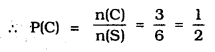Question 14.
One card is drawn from a well-shuffled deck of 52 cards. Find the probability of getting
(i) a king of red colour,
(ii) a face card
(iii) a red face card
(iv) the jack of hearts
(vi) the queen of diamonds.
Solution:
Total number of cards = 52
(i) P(One red king) = $$\frac{2}{52}=\frac{1}{26}$$
(ii) Number of a face card :
4 king, 4 queen, 4 jack = Total 12 cards
∴ P(1 face card) = $$\frac{12}{52}=\frac{3}{13}$$
(iii) One red colour face card = 6
∴ P(1 red face card) = $$\frac{6}{52}=\frac{3}{26}$$
(iv) P(Heart Jack) = $$\frac{1}{52}$$
(v) P(1 spade) = $$\frac{13}{52}=\frac{1}{4}$$
(vi) P(Diamond card) = $$\frac{1}{52}$$

Question 15.
Five cards— the ten, jack, queen, king and ace of diamonds, are well-shuffled with their face downwards. One card is then picked up at random.
(i) What is the probability that the card is the queen ?
(ii) If the queen is drawn and put aside, what is the probability that the second card picked up is
(a) an ace?
(b) a queen ?
Solution:
Five cards— the ten, jack, queen, king and ace of diamonds.
(i) Probability of getting queen = $$\frac{1}{5}$$
(ii) If the queen is drawn and put aside, the probability of getting other cards = 4.
(∵ 5 – 1 = 4)
∴ a) Probability that the card is ace = $$\frac{1}{4}$$
b) Probability that the card is queen = $$=\frac{0}{4}=0$$

Question 16.
12 defective pens are accidentally mixed with 132 good ones. It is not possible to just look at a pen and tell whether or not it is defective. One pen is taken out at random from this lot. Determine the probability that the pen taken out is a good one.
Solution:
Number of defective pens =12
Number of good pens =132
Total Number of pens = 12 + 132 = 144
The probability that the pen taken out is good one,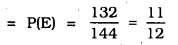Question 17.
(i) A lot of 20 bulbs contain 4 defective ones. One bulb is drawn at random from the lot. What is the probability that the bulb is defective ?
(ii) Suppose the bulb drawn in (i) is not defective and is not replaced. Now one bulb is drawn at random from the rest. What is the probability that this bulb is not defective ?
Solution:
Out of 20 bulbs, contain 4 defective ones.
(i) ∴ Probability of defective bulb = $$\frac{4}{20}$$
(ii) Out of 20, one bulb is drawn at random from the lot then remaining bulbs = 19.
Out of 19 bulbs, the bulbs which arc not defective = 19 – 4 = 15
∴ The probability that the bulb is not defective, P(E) = $$\frac{15}{19}$$

Question 18.
A box contains 90 discs which are numbered from 1 to 90. If one disc is drawn at random from the box, find the probability that it bears
(i) a two-digit number
(ii) a perfect square number
(iii) a number divisible by 5.
Solution:
Number of discs which are numbered from 1 to 30,
n(S) = 90
(i) A two-digit number :
Out of 90, one digit number = 9
∴ 2-digit numbers = 90 – 9 = 81
∴ 2-digit numbers, n(E) = 81(ii) A perfect square number :
1, 4, 9, 16, 25, 36, 49, 64, 81
∴ n(E) = 9(iii) A number divisible by 5 :
5, 10, 15, 20, 25, 30, 35, 40, 45, 50, 55, 60, 65, 70, 75, 80, 85, 90 .
∴ n(E) = 18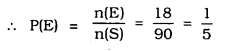Question 19.
A child has a die whose six faces show the letters as given below :The die is thrown once. What is the probbility of getting (i) A ?, (ii) D ?
Solution:
Number of faces in a die is 6.
∴ n(S) = 6
(i) The probability of getting face ‘A’:
∵ n(E) = 2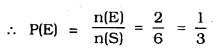(ii) The probbility of getting face ‘D’ is 1.
n(E) = 1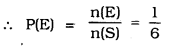Question 20.
Suppose you drop a die at random on the rectangular region as shown in the following figure. What is the probability that it will land inside the circle with diameter 1 m ?
(* Not from the examination point of view)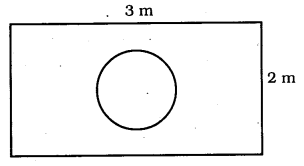Solution:
(i) Length of a rectnagular region is 3m.
Breadth of a rectangular region is 2m.
∴ Area of rectangle = length × breadth
= 3 × 2 = 6 sq.m.
(ii) A circle with diameter 1 m.
∴ Radius, r = $$\frac{1}{2}$$ m
Area of Circle = $$\pi r^{2}$$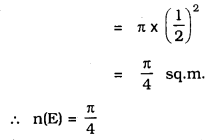∴ The probability that die will land inside the circle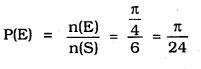Question 21.
A lot consists of 144 ball pens of which 20 are defective arid the others are good. Nuri will buy a pen if it is good, but will not buy If It is defective. The shopkeeper draws one pen at random and gives it to her. What is the probability that
(ii) She will not buy It?
Solution:
Total number of pens, n(S) = 144.
Number of defective pens = 20
∴ Number of good pens = 144 – 20
= 124
(i) Number of good pens Nun buys = 124
∴ n(E) = 124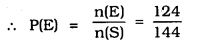(ii) Number of pens which Nuri do not buy = 20
∴ n(E) = 20Question 22.
Refer to Example 13.
(i) Complete the following table: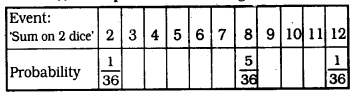(ii) A student argues that there are 11 possible outcomes 2, 3, 4, 5, 6, 7, 8, 9, 10, 11 and 12. Therefore each of them has a probability $$\frac{1}{11}$$. Do you agree with this argument ? Justify your answer.
Solution:

∵ The two dice are thrown together.
∴ Following are the possible outcomes :
(1, 1) ; (1, 2); (1, 3); (1, 4); (1, 5); (1, 6).
(2, 1) ; (2, 2); (2, 3); (2, 4); (2, 5); (2, 6).
(3, 1); (3, 2); (3, 3); (3, 4); (3, 5); (3, 6).
(4, 1) ; (4, 2); (4, 3); (4, 4); (4, 5); (4, 6).
(5, 1) ; (5, 2); (5, 3); (5, 4); (5, 5); (5, 6).
(6, 1) ; (6,.2); (6, 3); (6, 4); (6, 5); (6, 6).
∴ Total number of possible outcomes is 6 × 6 = 36
(i) (a) The sum on two dice is 3 for (1, 2) and (2, 1)
∴ Number of favourable outcomes = 2
⇒ P(3) = $$\frac{2}{36}$$

(b) The sum on two dice is 4 for (1, 3), (2, 2) and (3, 1).
∴ Number of favourable outcomes = 3
⇒ P(4) = $$\frac{3}{36}$$

(c) The sum on two dice is 5 for (1, 4), (2, 3), (3, 2) and (4,1)
∴ Number of favourable outcomes = 4
⇒ P(5) = $$\frac{5}{36}$$

(d) The sum on two dice is 6 for (1, 5), (2, 4), (3, 3), (4, 2) and (5,1)
∴ Number of favourable outcomes = 5
⇒ P(6) = $$\frac{5}{36}$$

(e) The sum on two dice is 7 for (1, 6), (2, 5), (3, 4), (4, 3), (5, 2) and (6,1)
∴ Number of favourable outcomes = 6
⇒ P(7) = $$\frac{62}{36}$$

(f) The sum on two dice is 9 for (3, 6), (4, 5), (5, 4) and (6, 3)
∴ Number of favourable outcomes = 4
⇒ P(9) = $$\frac{4}{36}$$

(g) The sum on two dice is 10 for (4, 6), (5, 5), (6,4)
∴ Number of favourable outcomes = 3
⇒ P(10) = $$\frac{3}{36}$$

(h) The sum on two dice is 11 for (5, 6) and (6,5)
∴ Number of favourable outcomes = 2
⇒ P(11) = $$\frac{2}{36}$$

Thus, the complete table is as follows:(ii) No. The number of all possible outcomes is 36 not 11.
∴ The argument is not correct.

Question 23.
A game consists of tossing a one rupee coin 3 times and noting its outcome each time. Hanif wins if all the tosses give the same result, i.e., three heads or three tails, and loses otherwise. Calculate the probability that Hanif will lose the game.
Solution:
Events when one rupee coin is tossed 3 times :
HHH, TTT, HHT, HTH, HTT, THT, TTH
∴ n(S) = 8
Out of these Event except HHH, TTT
Hanif loosing the game, n(E) = 6
(∵ 8 – 2 = 6)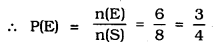Question 24.
A die is thrown twice. What is the probability that
(i) 5 will not come up either Urne?
(ii) 5 will come up at least once?
[Hint: Throwing a die twice and throwing two dice simultaneously are treated as the same experiment.)
Solution:
The possibility that one die is thrown:
n(S) = 6 × 6 = 36
(i) 5 will come up either time:
(1, 5), (2. 5), (3, 5), (4, 5), (5, 5), (6, 5), (5, 1), (5, 2), (5, 3) (5, 4), (5, 6)
∴ Possible events = 11.
∴ Probability that 5 will not come up either time : 36 – 11 = 25
n(E) = 25(ii) Possibility that 5 will come up at least ones: 11
∴ n(E) = 11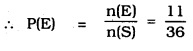Question 25.
Which of the following arguments are correct and which are not correct ? Give reasons for your answer.
(i) If two coins are tossed simultaneously there are three possible outcomes – two heads, two tails or one of each. Therefore, for each of these outcomes, the probability is $$\frac{1}{3}$$.
(ii) If a die is thrown, there are two possible outcomes — an odd number or an even number. Therefore, the probability of getting an odd number is $$\frac{1}{2}$$.
Solution:
(i) Given argument is not correct. Because, if two coins are tossed simultaneously then four outcomes are possible (HH, HT, TH, TT). So total outcomes is 4.
∴ The required probability = $$\frac{1}{4}$$.
(ii) Given argument is correct.
Since, total numebr of possible outcomes = 6
Odd numbers = 3 and even numbers = 3
So, favourable outcomes = 3 (in both the cases even or odd).
∴ Probability = $$\frac{3}{6}=\frac{1}{2}$$

We hope the given KSEEB SSLC Class 10 Maths Solutions Chapter 14 Probability Ex 14.1 will help you. If you have any query regarding Karnataka SSLC Class 10 Maths Solutions Chapter 14 Probability Exercise 14.1, drop a comment below and we will get back to you at the earliest.

## KSEEB SSLC Class 10 Science Solutions Chapter 5 Periodic Classification of Elements

KSEEB SSLC Class 10 Science Solutions Chapter 5 Periodic Classification of Elements are part of KSEEB SSLC Class 10 Science Solutions. Here we have given Karnataka SSLC Class 10 Science Solutions Chapter 5 Periodic Classification of Elements.

## Karnataka SSLC Class 10 Science Solutions Chapter 5 Periodic Classification of Elements

### KSEEB SSLC Class 10 Science Chapter 5 Intext Questions

Text Book Part II Page No. 25

Question 1.
Did Dobereiner’s triads also exist in the columns of Newlands’ Octaves? Compare and find out.
Yes, Dobereiner’s triads also exist in the columns of Newlands’ Octaves. One such column is Li, K, Na.

Question 2.
What were the limitations of Dobereiner’s classification?
Limitation of Dobereiner’s classification:
All known elements could not be classified into groups of triads on the basis of their properties.

Question 3.
What were the limitations of Newlands’ Law of Octaves?
Limitations of Newlands’ law of octaves:

1. It was not applicable throughout the arrangements. It was applicable up to calcium only. The properties of the elements listed after calcium showed no resemblance to the properties of the elements above them.
2. Elements discovered after Newlands’ octaves did not follow the law of octaves.
3. The position of cobalt and nickel in the group of the elements (F, Cl) of different properties could not be explained. Similarly, properties of iron also could not be explained.

Text Book Part II Page No. 29

Question 1.
Use Mendeleev’s Periodic Table to predict the formulae for the oxides of the following elements:
K, C, AI, Si, Ba.

1. K belongs to group 1. Therefore, the oxide will be K2O.
2. C belongs to group 4. Therefore, the oxide will be CO2.
3. Al belongs to group 3. Therefore, the oxide will be Al2O3.
4. Si belongs to group 4. Therefore, the oxide will be SiO2.
5. Ba belongs to group 2. Therefore, the oxide will be BaO.

Question 2.
Besides gallium, which other elements have since been discovered that were left by Mendeldev in his Periodic Table? (any two)
Scandium and Germanium.

Question 3.
What were the criteria used by Mendeleev in creating his Periodic Table?
Mendeleev considered the atomic mass of the elements as the unique criteria of the elements. He proposed that the chemical properties of elements are the periodic function of their atomic masses. And thus, he arranged the elements in the increasing order of their atomic masses.

Question 4.
Why do you think the noble gases are placed in a separate group?
Noble gases are very inert and they are different when compared to other elements. Hence, these are placed in separate group.

Text Book Part II Page No. 34

Question 1.
How could the Modern Periodic Table remove various anomalies of Mendeleev’s Periodic Table?

Various anomalies of Mendeleev’s Periodic Table are removed in the Modern Periodic Table as follows:

1. Elements are arranged in the increasing order of their atomic number in Modern Periodic Table, thus one element is placed in one position.
2. In Modern Periodic Table, there was no problem with the place of isotopes, as isotopes have the same atomic mass with different atomic numbers.
3. Elements having the same valence electrons are kept in the same group.
4. Elements having the same number of shells were put under the same period.
5. Position of hydrogen became clarified as it is kept in the group with the elements of same valence electrons.

Question 2.
Name two elements you would expect to show chemical reactions similar to magnesium. What is the basis for your choice?
Calcium and strontium are the two elements we would expect to show chemical reactions similar to magnesium. Because these resemble magnesium in chemical properties, All three elements have two valence electrons.

Question 3.
Name
(a) three elements that have a single electron in their outermost shells.
(b) two elements that have two electrons in their outermost shells.
(c) three elements with filled outermost shells.
(a) Lithium, sodium and potassium are the 3 elements which have single electron in their outermost orbit.
(b) Magnesium and calcium are the two elements which have 2 electrons in their outermost orbit.
(c) Noble gases such as Helium, Neon and Argon are the three elements whose outermost shells are completely filled.

Question 4.
Lithium, sodium, potassium are all metals that react with water to liberate hydrogen gas. Is there any similarity in the atoms of these elements? Helium is an unreactive gas and neon is a gas of extremely low reactivity. What, if anything, do their atoms have in common?
Yes. The atoms of all the three elements lithium, sodium and potassium have one electron in their outermost shells. Both helium (He) and neon (Ne) have filled outermost shells. Helium has a duplet in its K shell, while neon has an octet in its L shell.

Question 5.
In the Modern Periodic Table, which are the metals among the first ten elements?
Among the first ten elements, Lithium (Li) and Beryllium (Be) are metals.

Question 6.
By considering their position in the Periodic Table, which one of the following elements would you expect to have maximum metallic characteristic?
Ga Ge As Se Be
Berylium has maximum metallic characteristic.

### KSEEB SSLC Class 10 Science Chapter 5 Textbook Exercises

Question 1.
Which of the following statements is not a correct statement about the trends when going from left to right across the periods of Periodic Table.
(a) The elements become less metallic in nature.
(b) The number of valence electrons increases.
(c) The atoms lose their electrons more easily.
(d) The oxides become more acidic.
(c) The atoms lose their electrons more easily.

Question 2.
Element X forms a chloride with the formula XCl2, which is a solid with a high melting point. X would most likely be
in the same group of the Periodic Table as
(a) Na
(b) Mg
(c) AI
(d) Si
(b) Mg.

Question 3.
Which element has
(a) two shells, both of which are completely filled with electrons?
(b) the electronic configuration 2, 8, 2?
(c) a total of three shells, with four electrons in its valence shell?
(d) a total of two shells, with three electrons in its valence shell?
(e) twice as many electrons in its second shell as in its first shell?
(a) Neon
(b) Magnesium
(c) Silicon
(d) Boran
(e) Carbon

Question 4.
(a) What property do all elements in the same column of the Periodic Table as boron have in common?
(b) What property do all elements in the same column of the Periodic Table as fluorine have in common?
(a) All elements in Boron group have valency 3.
(b) All elements in fluorine group have valency 1.

Question 5.
An atom has electronic configuration 2, 8, 7.
(a) What is the atomic number of this element?
(b) To which of the following elements would it be chemically similar? (Atomic numbers are given in parentheses.)
N(7) F(9) P(15) Ar(18)
(a) Atomic Number is 17.
(b) N and P are chemically similar.

Question 6.
The position of three elements A, B and C in the Periodic Table are shown below –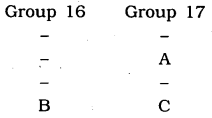(a) State whether A is a metal or non-metal.
(b) State whether C is more reactive or less reactive than A.
(c) Will C be larger or smaller in size than B?
(d) Which type of ion, cation or anion, will be formed by element A?
a) A is a Non metal.
b) C is less reactive comparing to A.
c) C is smaller in size comparing to A.
d) Cation is formed by A.

Question 7.
Nitrogen (atomic number 7) and phosphorus (atomic number 15) belong to group 15 of the Periodic Table. Write the electronic configuration of these two elements. Which of these will be more electronegative? Why?
Nitrogen (7): 2,5
Phosphorus (15): 2, 8, 5.
Since electronegativity decreases with moving from top to bottom in a group, thus nitrogen will be more electronegative.

Question 8.
How does the electronic configuration of an atom relate to its position in the Modern Periodic Table?
In the modern periodic table, elements having some electronic configuration are arranged in the same group.

Question 9.
In the Modern Periodic table, calcium (atomic number 20) is surrounded by elements with atomic numbers 12, 19, 21 and 38. Which of these have physical and chemical properties resembling calcium?
Atomic number 12 = 2, 8, 2
Atomic number 20 = 2, 8, 8, 2
Atomic Number 19 = 2,8, 8, 1
Atomic number 21 = 2, 8, 9, 2
Atomic number 38 = 2, 8, 18, 8, 2
Calcium will have similar physical and chemical properties as element with atomic numbers 12 and 38.

Question 10.
Compare and contrast the arrangement of elements in Mendeleev’s Periodic Table and the Modern Periodic Table.

Mendeleev s Periodic table:

1. Elements are arranged in the increasing order of their atomic mass.
2. This table has 8 groups and 6 periods. And each group is subdivided as an A and B.
3. In this table, Hydrogen has no position.
4. No position for isotopes, because in Mendeleev period these are not discovered.

Modern Periodic table:

1. Elements are arranged in the increasing order of their atomic number.
2. It has 18 groups and 7 periods.
3. Inert gases are placed in separate groups.
4. In this table, a zigzag line separates Metals from Non-metals.

(or)

Mendeleev’s Periodic table vs Modern Periodic table:

1. Elements are arranged in the increasing order of their atomic masses, while in Modern Periodic table elements are arranged in the increasing order of their atomic numbers.
2. There are a total of 7 groups (columns) and 6 periods (rows) while in Mendeleev’s’ Periodic Table, there are a total of 18 groups (columns) and 7 periods (rows).
3. Elements having similar properties were placed directly under one another, while in Mendeleev’s’ Periodic Table elements having the same number of valence electrons are present in the same group.
4. In Mendeleev’s Periodic Table the position of hydrogen could not be explained, while in Modern Periodic table hydrogen is placed above alkali metals.
5. No distinguishing positions for metals and non-metals in Mendeleev’s Periodic Table while in Modern Periodic Table metals are present at the left-hand side of the periodic table whereas nonmetals are present at the right-hand side.

### KSEEB SSLC Class 10 Science Chapter 5 Additional Questions and Answers

Fill in the blanks:

Question 1.
At present …….. elements are known to us.
118.

Question 2.
Law of triads was given by ……..
Dobereiner.

Question 3.
……. was introduced by Newlands’
Law of Octaves.

Question 4.
…….. was the most important contributor to the early development of a periodic table.
Mendeleev.

Question 5.
When Mendeleev started his work ……. elements were known.
63.

Question 6.
Hydrogen combines with metals and non-metals to form ………
Covalent compounds.

We hope the given KSEEB SSLC Class 10 Science Solutions Chapter 5 Periodic Classification of Elements will help you. If you have any query regarding Karnataka SSLC Class 10 Science Solutions Chapter 5 Periodic Classification of Elements, drop a comment below and we will get back to you at the earliest.

## KSEEB SSLC Class 10 Science Solutions Chapter 10 Light Reflection and Refraction

KSEEB SSLC Class 10 Science Solutions Chapter 10 Light Reflection and Refraction are part of KSEEB SSLC Class 10 Science Solutions. Here we have given Karnataka SSLC Class 10 Science Solutions Chapter 10 Light Reflection and Refraction.

## Karnataka SSLC Class 10 Science Solutions Chapter 10 Light Reflection and Refraction

### KSEEB SSLC Class 10 Science Chapter 10 Intext Questions

Text Book Part II Page No. 78

Question 1.
Define the principal focus of a concave mirror.
The number of rays parallel to the principal axis are falling on a concave mirror which meat at a point is called principal focus of the concave mirror.

Question 2.
The radius of curvature of a spherical mirror is 20 cm. What is its focal length?
R = 2f Here R = 20 cm
20 = 2f
∴ $$f=\frac { 20 }{ 2 } =10$$
∴ Focal length = 10 cm.

Question 3.
Name a mirror that can give an erect and enlarged image of an object.
Concave mirror.

Question 4.
Why do we prefer a convex mirror as a rear-view mirror in vehicles?
Because these mirrors are fitted on the sides of the vehicle, enabling the driver to see traffic behind him/her to facilitate safe driving.

Text Book Part II Page No. 81

Question 1.
Find the focal length of a convex mirror whose radius of curvature is 32 cm.
Radius of curvature, R = 32 cm
$$R=2f=\frac { R }{ 2 } =\frac { 32 }{ 2 } =16$$
∴ Convex mirror focal length is = 16cm

Question 2.
A concave mirror produces three times magnified (enlarged) real image of an object placed at 10 cm in front of it. Where is the image located?
$$M=\frac { Height\quad of\quad image }{ Height\quad of\quad object }$$
$$=\frac{h_{1}}{h_{0}}=\frac{-u}{v}$$
Let the height of object be h then height of image h = – 3h
$$=\frac{3 h}{h}=\frac{-v}{u}=\frac{v}{u}=3$$
∴ Distance of object, u = – 10 cm
v = 3 × (10) = – 30 cm
Here – sign indicates, image is real and it is 30 cm in front of concave mirror.

Text Book Part II Page No. 86

Question 1.
A ray of light traveling in air enters obliquely into water. Does the light ray bend towards the normal or away from the normal? Why?
Lightray bend towards normal. Because when a ray of light enters from rearer medium to denser medium, it changes its direction in the second medium.

Question 2.
Light enters from air to glass having refractive index 1.50. What is the speed of light in the glass ? The speed of light in vaccum is 3 108 ms-1
Refractive index, nm
$$=\frac { Velocity\quad of\quad light\quad in\quad vaccum\quad }{ Refractive\quad Index\quad of\quad glass }$$
$$=\frac{3 \times 10^{8}}{1.50}=2 \times 10^{8} \mathrm{m} / \mathrm{s}$$

Question 3.
Find out, from Tabel 10.3, the medium having highest optical density. Also find the medium with lowest optical density.
Diamond is having highest optical density.
Air is having lowest optical density.

Question 4.
You are given kerosene, turpentine and water. In which of these does the light travel fastest ? Use the information given in Table 10.3
Light travel faster in water because Refractive index of water is lesser than kerosene and turpentine.

Question 5.
The refractive index of diamond is 2.42. What is the meaning of this statement?
It means Ratio of velocity of light in air and velocity of air in diamond is 2.42.

Text Book Part II Page No. 94

Question 1.
Define 1 dioptre of power of a lens.
1 dioptre is the power of lens whose focal length is 1 metre 1 D = 1 m-1

Question 2.
A convex lens forms a real and inverted image of a needle at a distance of 50 cm from it. Where is the needle placed in front of the convex lens if the image is equal to the size of the object? Also, find the power of the lens.
Image of Needle is real and inverted means this is real image it is 2f
Image is at a distance of 50 cm
Hence needle is kept 50 cm in front of convex lens.
Distance of object, u = – 50 cm.
Distance of image v = 50 cm
Focal length f = ?
As per lens formula.f = 25 cm = 0.25 m
Power of the lens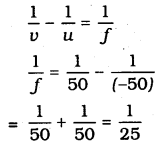Power of the lens P = + 4D.

### KSEEB SSLC Class 10 Science Chapter 10 Textbook Exercises

Question 1.
Which one of the following materials cannot be used to make a lens?
(a) Water
(b) Glass
(c) Plastic
(d) Clay
(d) Clay.

Question 2.
The image formed by a concave mirror is observed to be virtual, erect and larger than the object. Where should be the position of the object?
(a) Between the principal focus and the centre of curvature
(b) At the centre of curvature
(c) Beyond the centre of curvature
(d) Between the pole of the mirror and its principal focus.
(d) Between the pole of the mirror and its principal focus.

Question 3.
Where should an object be placed in front of a convex lens to get a real image of the size of the object?
(a) At the principal focus of the lens
(b) At twice the focal length
(c) At infinity
(d) Between the optical centre of the lens and its principal focus.
(b) At twice the focal length.

Question 4.
A spherical mirror and a thin spherical lens have each a focal length of -15 cm. The mirror and the lens are likely to be
(a) both concave.
(b) both convex.
(c) the mirror is concave and the lens is convex.
(d) the mirror is convex, but the lens is concave.
(a) both concave.

Question 6.
Which of the following lenses would you prefer to use while reading small letters found in a dictionary?
(a) A convex lens of focal length 50 cm.
(b) A concave lens of focal length 50 cm.
(c) A convex lens of focal length 5 cm.
(d) A concave lens of focal length 5 cm.
(c) A convex lens of focal length 5cm.

Question 7.
We wish to obtain an erect image of an object, using a concave mirror of focal length 15 cm. What should be the range of distance of the object from the mirror?
What is the nature of the image? Is the image larger or smaller than the object?
Draw a ray diagram to show the image formation in this case.
Distance of the object = o to 15 cm
Nature of image = virtual, erect and bigger than object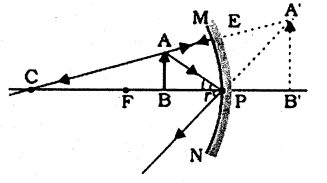Question 8.
Name the type of mirror used in the following situations.
(b) Side/rear-view mirror of a vehicle.
(c) Solar furnace.
(a) Concave mirrors are used as reflectors in headlights of cars. When a bulb is located at the focus of the concave mirror, the light rays after reflection from the mirror travel over a large distance as a parallel beam of high intensity.
(b) A convex mirror is used as a side/ rear-view mirror of a vehicle because,

• A convex mirror always forms an erect, virtual, and diminished image of an object placed anywhere in front of it.
• A convex mirror has a wider field of view than a plane mirror of the same size.

(c) Large concave mirrors are used to concentrate sunlight to produce heat in solar furnaces.

Question 9.
One-half of a convex lens is covered with a black paper. Will this lens produce a complete image of the object? Verify your answer experimentally. Explain your observations.
This lens gives full image, though one-half of this lens is covered with black paper as shown in below figure.As shown in figure light ray moves in half part and image is formed in another part of the lens.
If black paper is covered in lower part: Following figure explain thisQuestion 10.
An object 5 cm in length is held 25 cm away from a converging lens of focal length 10 cm. Draw the ray diagram and find the position, size and the nature of the image formed.
Height of object, h = 5 cm
Distance of object from converging lens u = 25 cm
Focal length of lens f = 10 cm
As per lens formula $$\frac{1}{v}-\frac{1}{u}=\frac{1}{f}$$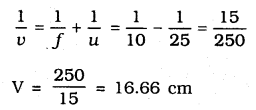Converging lens, $$\frac{h_{1}}{h_{0}}=\frac{v}{u}$$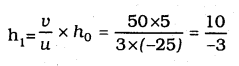= – 3.3 cm
Images is inverted and it is formed it is formed behind the lens about 16.7 cm. Its height is 3.3 cm.
Diagram is as follows: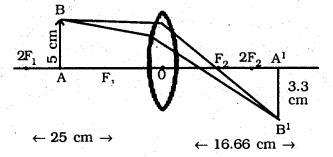Question 11.
A concave lens of focal length 15 cm forms an image 10 cm from the lens. How far is the object placed from the lens? Draw the ray diagram.
Focal length (F1) of concave lens
f = 15 cm
Image distance, v = – 10 cm
As per lens formulau = -30 cm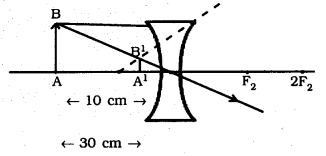Question 12.
An object is placed at a distance of 10 cm from a convex mirror of focal length 15 cm. Find the position and nature of the image.
Focal length of convex mirror,
f = +15 cm
Object distance, u = -10 cm
As per lens formula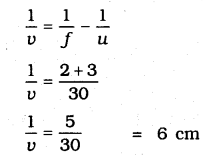Magnification $$=\frac{v}{u}=\frac{-6}{-10}=0.6$$
Virtual image is formed at the distance of 6 cm and it is erect.

Question 13.
The magnification produced by a plane mirror is +1. What does this mean?
The positive [+] sign of magnification [m] indicates that the image is virtual and erect. The magnification m = 1 indicates that the image is of the same size as the object. Thus, the magnification of +1 produced by a plane mirror means the image formed in a plane mirror is virtual, erect and of the same size as the object.

Question 14.
An object 5.0 cm in length is placed at a distance of 20 cm in front of a convex mirror of radius of curvature 30 cm. Find the position of the image, its nature and size.
Object distance, u = 20 cm
Height of object h = 5 cm
Radius of curvature R = 30 cm
R = 2f, f = 15 cm
As per mirror formulaMagnification $$=\quad \frac { Image\quad distance }{ Object\quad distance }$$
$$=\frac{-8.57}{-20}=0.428$$
Image is behind the mirror because magnification is positive
Magnification $$=\frac{\text { Image distance }}{\text { Object distance }}$$
$$=\frac{h^{1}}{h}$$
h1 = m × h = 0.428 × 5 = 2.14 cm

Question 15.
An object of size 7.0 cm is placed at 27 cm in front of a concave mirror of focal length 18 cm. At what distance from the mirror should a screen be placed so that a sharply focussed image can be obtained? Find the size and nature of the image.
Objective distance, u = 27 cm
Object height, h = 7 cm
Focal length, f = 30 cm
R = 2f, f = -18 cm
As per mirror formula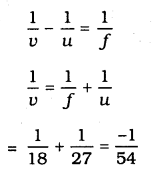Screen should be placed in front of mirror at the distance of = 54 cmNegative sign of magnification indicates image is real Magnification,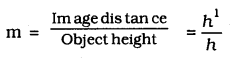h1 = 7 × (2) = -14 cm
Image is inverted because of negative sign.

Question 16.
Find the focal length of a lens of power – 2.0 D. What type of lens is this?
Power of lens, P $$=\frac{1}{f}$$
P = -2D
f $$=\frac{-1}{2}=-0.5 \mathrm{cm}$$

Question 17.
A doctor has prescribed a corrective lens of power +1.5 D. Find the focal length of the lens. Is the prescribed lens diverging or converging?
Power of lens, P $$=\frac{1}{f}$$
P = 1.5 D
F $$=\frac{1}{1.5}=\frac{10}{15}=0.66 \mathrm{m}$$
This is converging lens means convex lens.

### KSEEB SSLC Class 10 Science Chapter 10 Additional Questions and Answers

Question 1.
What are spherical mirrors?
Mirrors, whose reflecting surfaces are spherical, are called spherical mirrors.

Question 2.
What is pole of the mirror?
The centre of the reflecting surface of a spherical mirror is a point called the pole.

Question 3.
What is principal axis?
The line passing through the pole and the centre of curvature of a spherical mirror is called principal axis.

Question 4.
Draw a ray diagram of concave mirror and convex mirror.